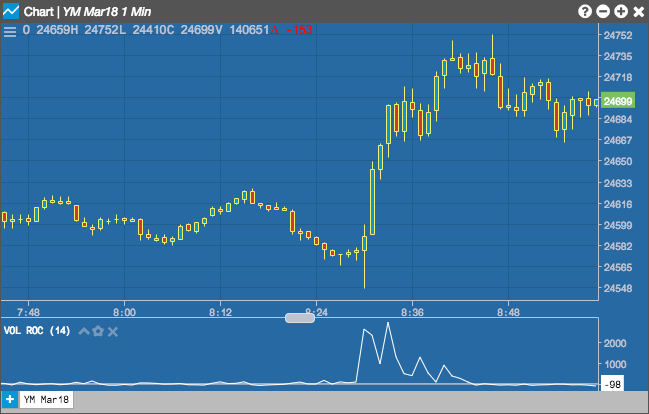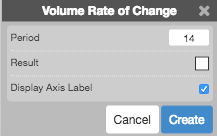Charts

# Volume Rate of Change

The Volume Rate of Change indicator measures the rate of change in the volume over a period of time.## Configuration Options• Period: Number of bars to use in the calculations.
• Color Selectors: Colors to use for graph elements.
• Display Axis Label: Whether to display the most recent value on the Y axis.

## Formula

$VROC = \frac{Volume - Volume_\text{previous-period}}{Volume_\text{previous-period}} \times 100$